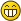# MyBB Community Forums

You're currently viewing a stripped down version of our content. View the full version with proper formatting.
Pages: 1 2 3

Like in Wikipedia where you push "Random Article". Members would push Random Thread and it takes you to a, Well a random thread from the forum.Thank you.
You would need a plugin - if you want the link to work anyway.
(2012-09-22, 05:15 PM)Omar G. Wrote: [ -> ]This may work:

Thanks but that's a bit old, I gave a quick try but got SQL Error: 1146
Maybe if you post the full error message we can help you to get it working.
Oops sorry didn't think of that.Open plugin file and find;
``\$threadcount = \$db->fetch_field(\$db->simple_select(TABLE_PREFIX.'threads', 'COUNT(*) AS threadcount', \$where), 'threadcount');``
and Change it into;
``\$threadcount = \$db->fetch_field(\$db->simple_select('threads', 'COUNT(*) AS threadcount', \$where), 'threadcount');``

Next, find;
``\$rndtid = \$db->fetch_field(\$db->simple_select(TABLE_PREFIX.'threads', 'tid', \$where, array('limit' => 1, 'limit_start' => \$rndnum)), 'tid');``
and Change it into;
``\$rndtid = \$db->fetch_field(\$db->simple_select('threads', 'tid', \$where, array('limit' => 1, 'limit_start' => \$rndnum)), 'tid');``
(2012-09-22, 09:09 PM)Yaldaram Wrote: [ -> ]Open plugin file and find;
``\$threadcount = \$db->fetch_field(\$db->simple_select(TABLE_PREFIX.'threads', 'COUNT(*) AS threadcount', \$where), 'threadcount');``
and Change it into;
``\$threadcount = \$db->fetch_field(\$db->simple_select('threads', 'COUNT(*) AS threadcount', \$where), 'threadcount');``

Next, find;
``\$rndtid = \$db->fetch_field(\$db->simple_select(TABLE_PREFIX.'threads', 'tid', \$where, array('limit' => 1, 'limit_start' => \$rndnum)), 'tid');``
and Change it into;
``\$rndtid = \$db->fetch_field(\$db->simple_select('threads', 'tid', \$where, array('limit' => 1, 'limit_start' => \$rndnum)), 'tid');``

Thanks Yaldaram, close it works when logged in, but for guests I get a blank page with "There are no threads on this board."
Find:
``\$where = "closed NOT LIKE 'moved|%' AND visible <> 0\$us";``

Replace with:
``\$where = "closed NOT LIKE 'moved|%' AND visible!='0'\$us";``
(2012-09-23, 01:45 AM)Omar G. Wrote: [ -> ]Find:
``\$where = "closed NOT LIKE 'moved|%' AND visible <> 0\$us";``

Replace with:
``\$where = "closed NOT LIKE 'moved|%' AND visible!='0'\$us";``

Hello, Didn't work still got the same result. Blank page with "There are no threads on this board."
Pages: 1 2 3